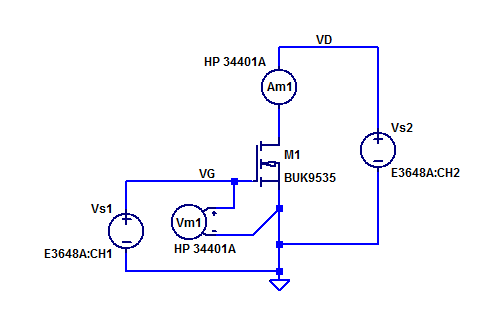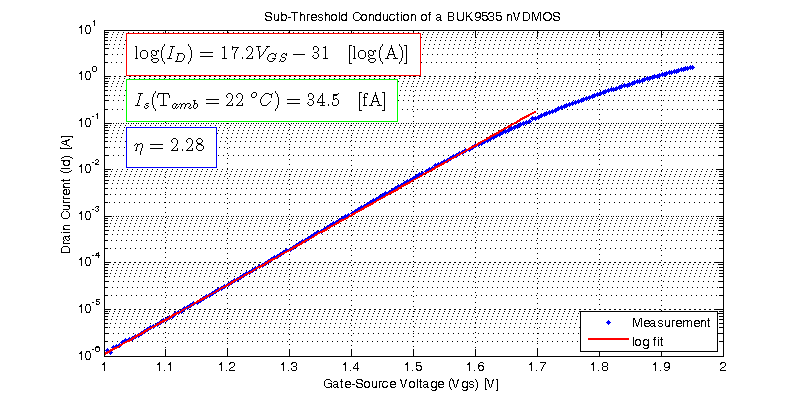# Sub-Threshold Conduction of a Power MOSFET

The banner line description of the BUK9535 power MOSFET is given as,

Logic level N-channel enhancement mode Field-Effect Transistor (FET) in a plastic package using TrenchMOS technology

In the sub-threshold region we can fit the drain current to the following basic exponential model,

$$I_D =I_S \exp\left( \dfrac{V_{GS}}{\eta V_T}\right)$$

## Measurement Setup

Bench supply $$V_{s1}$$ provides the gate-source bias potential $$V_{GS}$$. Voltmeter $$V_{m1}$$ records the effective gate-source potential at the terminals of $$M_1$$. Bench supply $$V_{s2}$$ biases the drain of $$M_1$$ at $$\simeq 5$$ VDC. With $$V_D$$ = 5, $$M_1$$ is biased into the saturation region for a diverse range of gate drive potentials. A schematic of the test setup is shown in the figure below.Ammeter $$A_{m1}$$ will present a small burden voltage in series with supply $$V_{s1}$$. $$A_{m1}$$ employs a $$5\Omega$$ current shunt resistor for its $$10 \& 100$$ mA current ranges. Worst case burden in the sub-threshold region is,

$$V_{BRD} = (0.1)(5) = 500 \text{ mV}$$

With a 100 mA of drain current the drain potential will decline from 5V to 4.5V a 10% reduction in drain bias. Fortunately $$M_1$$ has a modest $$\lambda$$ in the sub-threshold region.

## Measurement Results

The results of sweeping $$V_{GS}$$ of a sample DUT at a fixed $$V_{DS}$$ of 5 V is shown in the figure below.The log fit results in the following exponential function of $$V_{GS}$$,

$$I_D =34.5\text{ fA } \exp\left( \dfrac{V_{GS}}{58 \text{ mV}}\right)$$

Every 10x increase in drain current in the sub-threshold region requires an additional gate drive voltage of,

$$\Delta V_{GS} = \eta V_T \log(10) = 134 \text{ mV/dec}$$

## 1 thought on “Sub-Threshold Conduction of a Power MOSFET”

1.Ss says:

HI

This site uses Akismet to reduce spam. Learn how your comment data is processed.